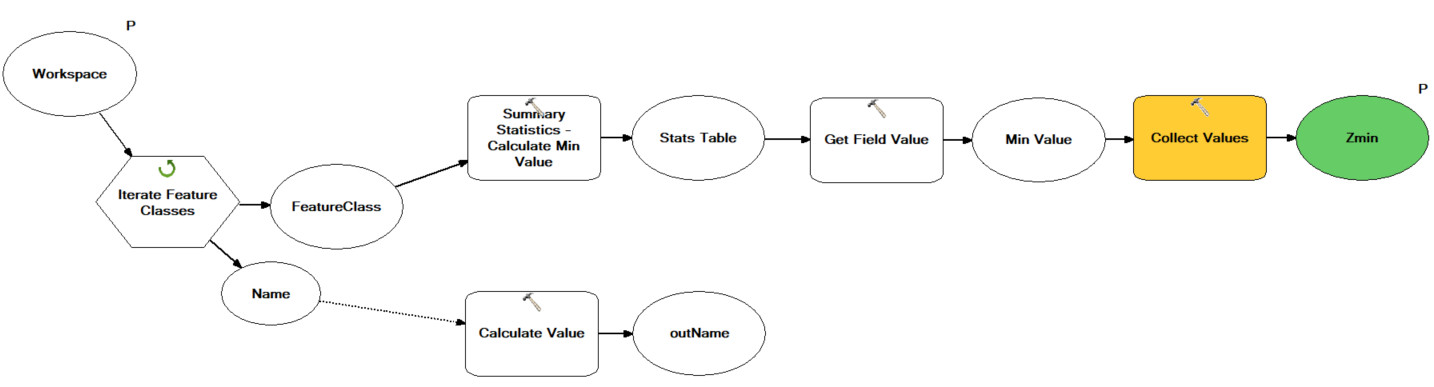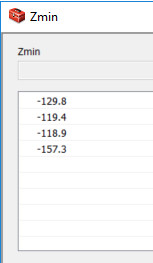# Calculate value from collected values not working

268
2
01-15-2019 12:34 AMNew Contributor II

I am building up a nested model that iterates through N feature classes (point shapefiles) in order to calculate statistics for field X and get the minimum value for each feature class. So far so good.

The iteration includes the model tool Get Field Value to extract the minimum value from the summary statistics and the Collect Values Tool to collect the values (Zmin, see figure below). It works properly.Next step would be to extract the minimum out of the N collected values (Zmin) and use it as a parameter in the main model:The output of the nested model (Zmin) is returned as multiple values:I have tried to use the Calculate Value Tool applying the following function:

Expression:

lowest("%Zmin%")

Code Block:

def lowest(Zmin):
Zmin.replace(";",",")
Zmin.split(',')
LowestLine = min(Zmin)
return LowestLine

Data Type: Double

It only returns '-', thus something is obviously wrong in the code but I cannot figure out what, due to my limited Python skills. Any help would be very much appreciated! Thanks in advance.

Tags (4)
2 RepliesbyMVP Notable Contributor

You were almost correct! Your function should be:

``````def lowest(Zmin):
l = Zmin.split(";") # splits string by semi-colon to return a LIST
lowestline = min(l)
return lowestline‍‍‍‍‍‍‍‍‍‍‍‍‍‍‍‍``````

This will return -118.9. If you wanted to return -157.3 then you use the max() function.byEsri Esteemed Contributor

I think you would first have to convert the strings in the newly created list to float in order to use the min correctly otherwise you are sorting the list alphabetically. So try this:

``````def lowest(Zmin):
lst = Zmin.split(";") # splits string by semi-colon to return a LIST
lst = [float(l) for l in lst]
lowestline = min(lst)
return lowestline
‍‍‍‍‍``````